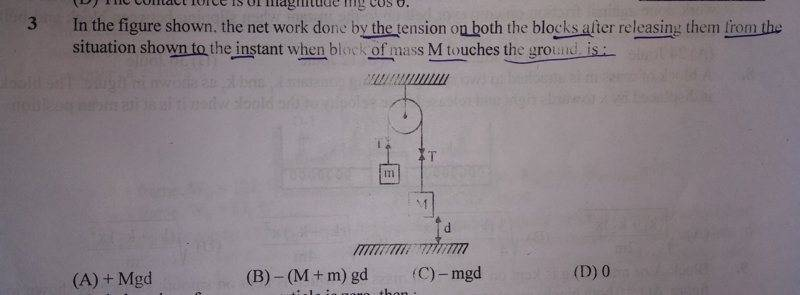# Intuitive explanation of why work done by tension is 0?

• navneet9431
In summary, the conversation discusses the concept of net work done by tension in a system involving two masses connected by a rope and a pulley. The solution involves using equations and the intuitive explanation given is that for a constant force, work is equal to force times displacement, and in a system with equal and opposite forces and equal displacements, the net work done by tension would be zero. This can be generalized to variable forces using integrals.f

Gold Member

## Homework Statement## Homework Equations

Work Done=Force*Displacement in the direction of the force

## The Attempt at a Solution

I tried to solve the problem this way,
I wrote these equations for the two masses,
T-mg=ma...(i)
Mg-T=Ma...(ii)
From (i)&(ii), T=[M(g-a)+m(a+g)]/2
and then I would multiply them with the displacement to get the net work done 0.
So this is how I solved it mathematically.
Can you please provide an intuitive explanation of why the net work done by tension would be zero?
I will be thankful for help!

#### Attachments

Can you please provide an intuitive explanation of why the net work done by tension would be zero?
Suppose you have two tug-of-war teams pulling on a rope and both teams are at a standstill. What work is being done?

But the pulley is not standstill.right?
Suppose you have two tug-of-war teams pulling on a rope and both teams are at a standstill. What work is being done?

But the pulley is not standstill.right?
OK, suppose one team walks forward at 2mph and the other team walks backwards at 2mph, it which case the tension is unchanged. How much work does the tension do?

provide an intuitive explanation of why the net work done by tension would be zero?
For a constant force, work = force times displacement.
In a tug of war, equal and opposite forces, equal displacements. Fd+(-F)d=0.
For the pulley set up in the diagram, equal forces, equal and opposite displacements. F.d+F(-d)=0.
Easy to generalise to variable forces using integrals.

•navneet9431
For a constant force, work = force times displacement.
In a tug of war, equal and opposite forces, equal displacements. Fd+(-F)d=0.
For the pulley set up in the diagram, equal forces, equal and opposite displacements. F.d+F(-d)=0.
Easy to generalise to variable forces using integrals.
Thanks!
It was easy to understand.